﻿ 10 Things You Need to Know Before Getting Started with TensorFlow

# 10 Things You Need to Know Before Getting Started with TensorFlowThough I took two college electives related to artificial intelligence (AI) and have used quite a few machine learning (ML) libraries, I am by no means a ML developer. However, like many developers nowadays, I am extremely curious about ML and TensorFlow, a popular library brought up in many conversations surrounding ML. What exactly is it?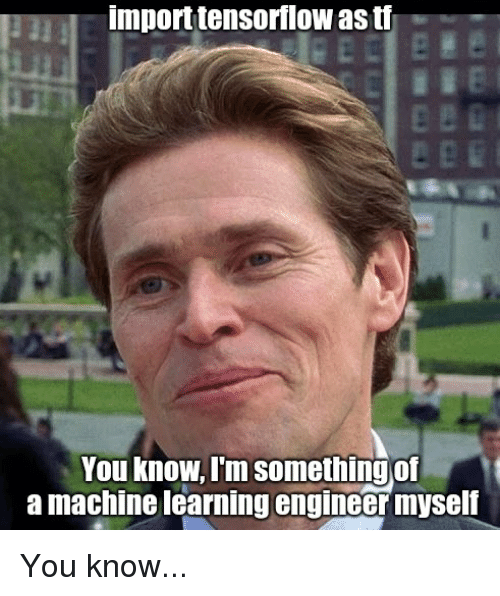### What is TensorFlow?

TensorFlow is an open source library released by Google Brain (now Google AI) in 2015 to make it easier for developers to build, train, and generally work with deep learning models and data to make different types of predictions. You can solve tasks like image classification, natural language processing, generate music as in this Twilio post, and more.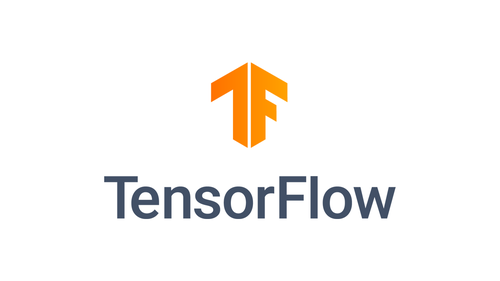I began playing around with TensorFlow a few weeks ago and though it's been fun and I've learned a lot, here are ten things I wish I'd known before using it.

### 10 Things You Need to Know before Getting Started With TensorFlow

1. There are many different ways to use TensorFlow. For one, it supports lots of languages. The most commonly-used one is probably Python, followed by JavaScript. Additionally there is support for Swift, C, Go, Java, Haskell, C#, Go, and more. This blog post includes some brief code snippets in Python which you can install with `pip` here. Make sure the version number is 2.0: a lot of TensorFlow code online is still in older versions.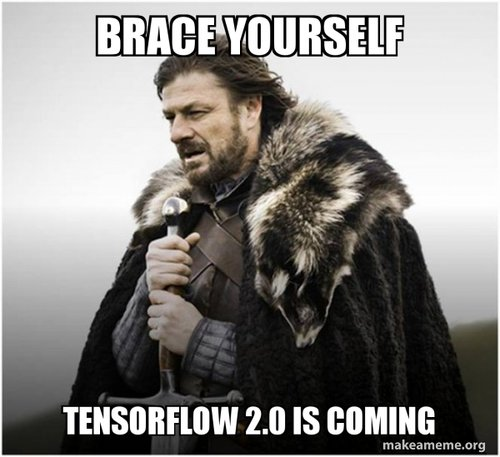There's also other TensorFlow tools to make your machine learning development easier. TensorFlow Serving is a flexible serving system that lets developers seamlessly serve, or work, their machine learning models (TensorFlow and other) once they're trained. You could deploy a new model version while TensorFlow Serving nicely finishes current requests and then begin to serve new requests with the new model.There's also the TensorFlow Lite deep learning framework for deploying models on mobile and embedded or IoT devices. It starts with training ML models on TensorFlow then converts them to Lite models to work on those devices. If I had more time, I'd love to look at ways to use both TensorFlow Lite and Twilio Programmable Wireless!2. Be mindful of the differences between TensorFlow versions. A lot of the code you find online will still be TensorFlow 1.x, and 1.x is very different from the most recent TensorFlow 2.x. TensorFlow 1.x had placeholders which are like variables that don't have a value attached to them at first, but can have one added to it later. As a heads-up, these are no longer around in TensorFlow 2.0! TensorFlow 2.0 did notably add eager execution mode so each node is immediately executed after definition and can be invoked with `tf.eager_execution()`. This eager execution now makes TensorFlow an imperative programming environment ("define-by-run") that performs operations straight away, without building pre-constructed graphs with `Session.run()`. No longer are computational graphs that run later returned: now operations return actual values letting you write fewer lines of code. It also makes it easier to debug, providing both a better user interface (UI) and more natural control flow with Python statements instead of graph control flow.

3. TensorFlow doesn't abstract as many of the hard parts of programming as most APIs do. I personally find that APIs like Twilio and the Dad Jokes API make my life easier by nicely wrapping the hardest parts and having easy-to-understand documentation. Though TensorFlow does quite a bit of heavy lifting with regards to training machine learning models (which is not easy to do), it's very low-level and you still need to understand quite a bit of machine learning, which leads us to #4:

4. You still need to (mostly) understand ML. Say you want to train and evaluate a model's accuracy. You may have some code like this (this is not copy-and-pasteable):

``````model = Sequential([
input_shape=(224, 224, 3)),
MaxPooling2D(),
MaxPooling2D(),
MaxPooling2D(),
Flatten(),
Dense(512, activation='relu'),
Dense(1, activation='sigmoid')
])
loss='binary_crossentropy',
metrics=['accuracy'])
history = model.fit_generator(
train_data_gen,
steps_per_epoch=total_train // batch_size,
epochs=epochs,
validation_data=val_data_gen,
validation_steps=total_val // batch_size
)
``````

You could then see something like this in your terminal: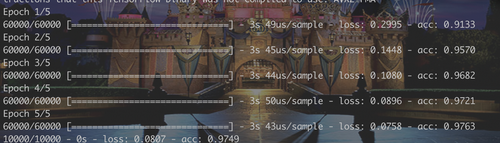An epoch is a full iteration over the training data by the machine learning algorithm. Relatedly, you would need to understand different models (sequential versus functional), loss functions (ways of evaluating how well an algorithm models data), cross-entropy loss (a loss function that returns output of a value between zero and one), activation functions for deep neural networks, padding (the amount of pixels added to an image processed by the kernel of a Convolutional Neural Network, it can be same or valid), and more. In short, be prepared for TensorFlow's learning curve.5. How did TensorFlow get its name?

All computations in TensorFlow involve tensors, which are multidimensional data arrays that neural networks perform computations on. Tensors also include scalars, vectors, or matrices of n-dimensions representing all types of data.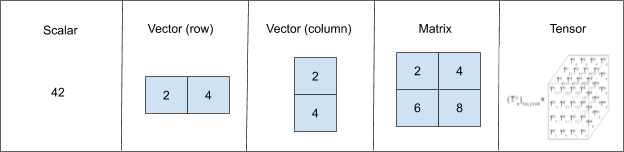A scalar is a single number with only magnitude, or size. A vector (both row and column vectors) is a 1D array of numbers with both magnitude and direction. Each item in a vector can be referenced by one index. A matrix is a 2D array of numbers whose numbers must be referenced by two indices. Finally, though a tensor is an array with more than two axes, it is also used to generalize or encompass scalors, vectors, and matrices. Each value in a tensor holds identical data types with a known or semi-known shape which is the dimensionality of the matrix or array. Each tensor is an object with three properties: a unique `name`, `shape` (dimension), and `dtype` (data type). `dtype` is common in scientific computing libraries like `NumPy` and `Scikit`.We will see more tensors in #6:

6. Constants and variables in TensorFlow are different and can be more complicated than need be. In TensorFlow you can create a constant tensor, or a node whose values does not change. In Python, a tensor constant of one dimension could be made with this:

``````import tensorflow as tf
x = tf.constant(7.0, dtype=tf.float32)
y = tf.constant([1,2,3,4])
``````

`x` is a constant of type 32-bit single-precision floating-point and `y` is a constant array. If you were to print these constants out you'd see that the output is an object of type tensor: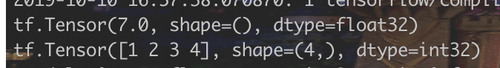There are two constants, the first is of shape [] (a scalar which is a rank 0 tensor) and the second is of shape  (a vector which is a rank 1 tensor.)

 Rank Object 0 scalar 1 vector 2 matrix ≥ 3 tensor

You can also perform computations on constants like so:

``````thirdNode = tf.add(5,4)
print(thirdNode)
``````

This would print out: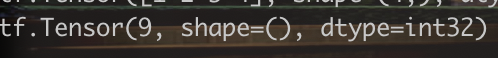WOW such complexity! How does TensorFlow handle other types, like variables? Variables represent nodes whose values can change. `tf.Variable` can be used to store the state of the data for trainable variables like weights and biases:

``````a = tf.Variable(3.14)
``````

You could also pass it optional parameters like `name` (ie. "a") and `dtype` (ie. "tf.float32") like this:

``````a=tf.Variable(3.14, dtype=tf.float32,name="a")
``````

To print out the value, we'd need to initialize all variables before the other model operations and since we build a model first, we have to run the initializer in the session first. The following cleanly initializes the `a` variable.

``````with tf.compat.v1.Session() as sess:
print(sess.run(a.initializer))
print(sess.run(a))
``````

It took me a few minutes to wrap my head around running the initializer first, and it's weird because it can be written in different ways! (Code is wild like that, I know.)

7. There are twenty-three data types tensors can take up. These data types gave me flashbacks to taking Discrete Math: as if signed (8-, 16-, 32-, and 64-bit) and unsigned (8- and 16-bit) integers, byte arrays, floating point numbers, and booleans were not enough, tensors can also take up quantized integers (signed and unsigned of varying bits), half-, single-, and double-precision floating points as well as truncated floating points and 64-bit single precision complex and 128-bit double-precision complex, to name a few. Yes, I do appreciate the variety of options and understand that these are helpful for complex achine learning models; however, they are mostly unnecessary for my personal ML projects and use cases.

8. Gathering and preparing training data can be a pain. Say you're performing image classification and need a lot of images to train your TensorFlow model on. You could gather a lot of images in Python like this:

``````import tensorflow as tf
import pathlib
fname='flower_photos', untar=True)
data_dir = pathlib.Path(data_dir)
``````

`googleimagesdownload --keywords 'panda' --limit 200 --size medium \`
`--chromedriver ./chromedriver --format png`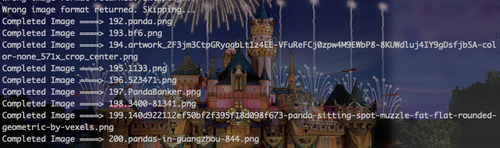Then, as if that wasn't enough work, each image has a different name! You should then rename each image with a number before labeling them manually. labelImg is one tool that helps you do annotate large datasets of images.This saves label (.xml) files in the common Pascal VOC format, the XML file format used by ImageNet, a popular academic dataset to train an image recognition system in ML.

9. There are a few alternatives to TensorFlow. One more commonly-used alternative is PyTorch, a deep learning framework developed by Facebook. PyTorch is based on the Torch scientific computing library and is also written in C++ with Python and C++ front-ends. PyTorch is more Pythonic than TensorFlow and lets developers easily build deep neural networks. While TensorFlow provides static graphs and the ability to use dynamic models with eager execution, PyTorch has dynamic computational graphs you can change during runtime. Then there's also Keras, a more high-level neural network API built in Python that is now a part of TensorFlow. It is more user-friendly because it's built in Python (whereas the core of TensorFlow is written in highly-optimized C++). However, because Keras is not a library on its own, it's unfair to compare it with TensorFlow.10. There are so many different use cases for TensorFlow. You can use it for voice recognition, sentiment analysis, language detection, text summarization, image recognition, video detection, time series, and more. The sky is the limit!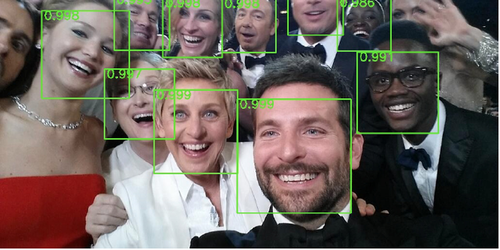In spite of some complications, TensorFlow offers great GPU and different hardware/OS environment support. Its flexible and modular design makes it easy to work with a lot of data, work in different languages (or train and execute models all in the browser with TensorFlow.js!), move models across CPU, GPU, or TPU processors with only a few code modifications, or perform not just machine learning and deep learning algorithms, but also statistical and general computational models. Lastly, there's great community support and because it's backed by Google, there's always work being done to improve it (so it's often evolving, which can be both good and bad.)

### Conclusion

After taking two AI-related computer science electives, I considered myself a ML enthusiast; now after using TensorFlow, I feel more like a ML developer. So what are you waiting for? Stay tuned to the Twilio blog for more TensorFlow content. Until then, let me know in the comments or online what you'll build with TensorFlow.

GitHub: elizabethsiegle

email: lsiegle@twilio.com

Rate this post
Authors
•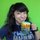Lizzie Siegle
Not ready yet? Talk to an expert.

## Thanks for rating this post!

We are always striving to improve our blog quality, and your feedback is valuable to us. How could this post serve you better?